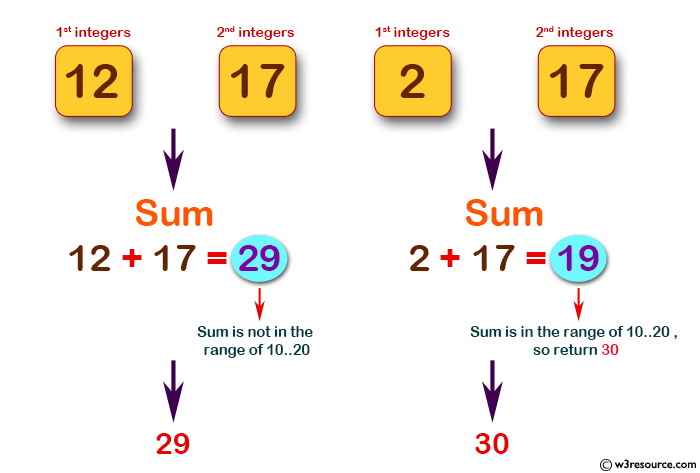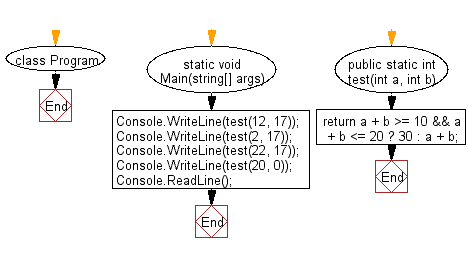﻿ C# - Compute the sum of the two given integers - w3resource# C# Sharp Basic Algorithm Exercises: Compute the sum of the two given integers

## C# Sharp Basic Algorithm: Exercise-40 with Solution

Write a C# Sharp program to compute the sum of the two given integers. If the sum is in the range 10..20 inclusive return 30.

Pictorial Presentation:Sample Solution:-

C# Sharp Code:

``````using System;
using System.Linq;
namespace exercises
{
class Program
{
static void Main(string[] args)
{
Console.WriteLine(test(12, 17));
Console.WriteLine(test(2, 17));
Console.WriteLine(test(22, 17));
Console.WriteLine(test(20, 0));
}
public static int test(int a, int b)
{
return a + b >= 10 && a + b <= 20 ? 30 : a + b;
}

}
}
```
```

Sample Output:

```29
30
39
30```

Flowchart:C# Sharp Code Editor:

Improve this sample solution and post your code through Disqus

What is the difficulty level of this exercise?

Test your Programming skills with w3resource's quiz.

﻿